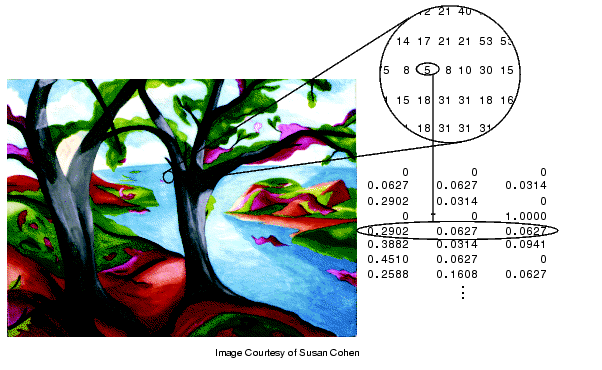Image Processing Toolbox User's GuideIndexed Images

An indexed image consists of an array, called `X` in this documentation, and a colormap matrix, called `map`. The pixel values in the array are direct indices into a colormap.

The colormap matrix `i`s an m-by-3 array of class `double` containing floating-point values in the range [0,1]. Each row of `map` specifies the red, green, and blue components of a single color. An indexed image uses direct mapping of pixel values to colormap values. The color of each image pixel is determined by using the corresponding value of `X` as an index into `map`.

A colormap is often stored with an indexed image and is automatically loaded with the image when you use the `imread` function. After you read the image and the colormap into the MATLAB workspace as separate variables, you must keep track of the association between the image and colormap. However, you are not limited to using the default colormap--you can use any colormap that you choose.

The relationship between the values in the image matrix and the colormap depends on the class of the image matrix. If the image matrix is of class `single` or `double`, it normally contains integer values 1 through p, where p is the length of the colormap. the value 1 points to the first row in the colormap, the value 2 points to the second row, and so on. If the image matrix is of class `logical`, `uint8` or `uint16`, the value 0 points to the first row in the colormap, the value 1 points to the second row, and so on.

The following figure illustrates the structure of an indexed image. In the figure, the image matrix is of class `double`, so the value 5 points to the fifth row of the colormap.Pixel Values Index to Colormap Entries in Indexed ImagesBinary Images Grayscale Images